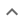•# Reducing fractions to their lowest terms

We think you wrote:

###This solution deals with reducing fractions to their lowest terms.

Solution found(a^2-4a-9)/(a)

## Step by Step Solution## Step  1  :

            9
Simplify   —
a


#### Equation at the end of step  1  :

        9
(a -  —) -  4
a


## Step  2  :

#### Rewriting the whole as an Equivalent Fraction :

Subtracting a fraction from a whole

Rewrite the whole as a fraction using  a  as the denominator :

          a     a • a
a =  —  =  —————
1       a


Equivalent fraction : The fraction thus generated looks different but has the same value as the whole

Common denominator : The equivalent fraction and the other fraction involved in the calculation share the same denominator

#### Adding fractions that have a common denominator :

Adding up the two equivalent fractions
Add the two equivalent fractions which now have a common denominator

Combine the numerators together, put the sum or difference over the common denominator then reduce to lowest terms if possible:

 a • a - (9)     a2 - 9
———————————  =  ——————
a            a


#### Equation at the end of step  2  :

  (a2 - 9)
———————— -  4
a


## Step  3  :

#### Rewriting the whole as an Equivalent Fraction :

Subtracting a whole from a fraction

Rewrite the whole as a fraction using  a  as the denominator :

         4     4 • a
4 =  —  =  —————
1       a


#### Trying to factor as a Difference of Squares :

Factoring:  a2 - 9

Theory : A difference of two perfect squares,  A2 - B2  can be factored into  (A+B) • (A-B)

Proof :  (A+B) • (A-B) =
A2 - AB + BA - B2 =
A2 - AB + AB - B2 =
A2 - B2

Note :  AB = BA is the commutative property of multiplication.

Note :  - AB + AB equals zero and is therefore eliminated from the expression.

Check : 9 is the square of 3
Check :  a2  is the square of  a1

Factorization is :       (a + 3)  •  (a - 3)

#### Adding fractions that have a common denominator :

Adding up the two equivalent fractions

 (a+3) • (a-3) - (4 • a)     a2 - 4a - 9
———————————————————————  =  ———————————
a                     a


#### Trying to factor by splitting the middle term

Factoring  a2 - 4a - 9

The first term is,  a2  its coefficient is  1 .
The middle term is,  -4a  its coefficient is  -4 .
The last term, "the constant", is  -9

Step-1 : Multiply the coefficient of the first term by the constant   1 • -9 = -9

Step-2 : Find two factors of  -9  whose sum equals the coefficient of the middle term, which is   -4 .

 -9 + 1 = -8 -3 + 3 = 0 -1 + 9 = 8

Observation : No two such factors can be found !!
Conclusion : Trinomial can not be factored

## Final result :

  a2 - 4a - 9
———————————
a